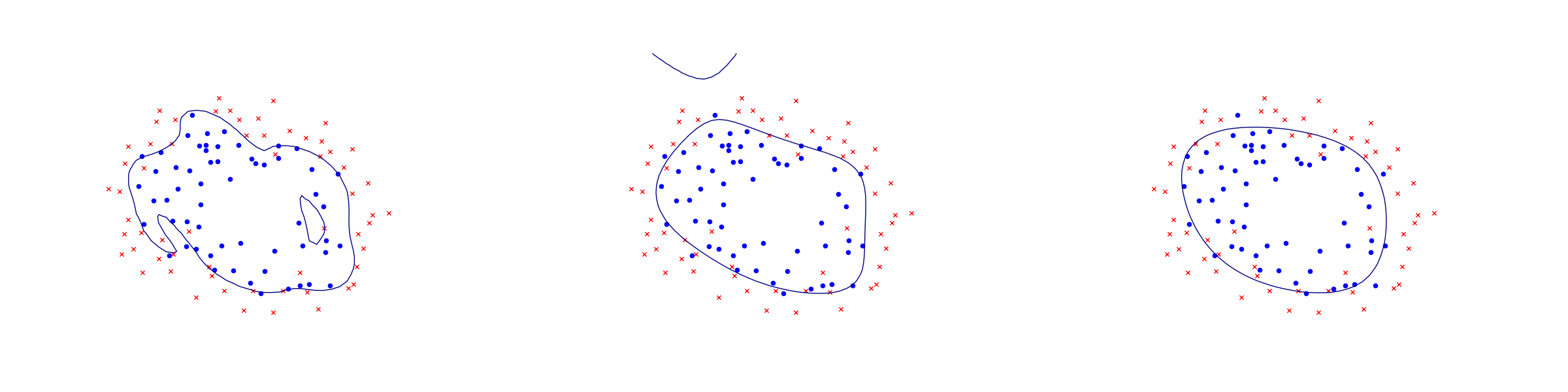# 10.3 Logistic regression¶

Logistic regression is an example of a binary classifier, where the output takes one two values 0 or 1 for each data point. We call the two values classes.

Formulation as an optimization problem

Define the sigmoid function

$S(x)=\frac{1}{1+\exp(-x)}.$

Next, given an observation $$x\in\real^d$$ and a weights $$\theta\in\real^d$$ we set

$h_\theta(x)=S(\theta^Tx)=\frac{1}{1+\exp(-\theta^Tx)}.$

The weights vector $$\theta$$ is part of the setup of the classifier. The expression $$h_\theta(x)$$ is interpreted as the probability that $$x$$ belongs to class 1. When asked to classify $$x$$ the returned answer is

$\begin{split}x\mapsto \begin{cases}\begin{array}{ll}1 & h_\theta(x)\geq 1/2, \\ 0 & h_\theta(x)<1/2.\end{array}\end{cases}\end{split}$

When training a logistic regression algorithm we are given a sequence of training examples $$x_i$$, each labelled with its class $$y_i\in \{0,1\}$$ and we seek to find the weights $$\theta$$ which maximize the likelihood function

$\prod_i h_\theta(x_i)^{y_i}(1-h_\theta(x_i))^{1-y_i}.$

Of course every single $$y_i$$ equals 0 or 1, so just one factor appears in the product for each training data point. By taking logarithms we can define the logistic loss function:

$J(\theta) = -\sum_{i:y_i=1} \log(h_\theta(x_i))-\sum_{i:y_i=0}\log(1-h_\theta(x_i)).$

The training problem with regularization (a standard technique to prevent overfitting) is now equivalent to

$\min_\theta J(\theta) + \lambda\|\theta\|_2.$

This can equivalently be phrased as

(10.21)$\begin{split}\begin{array}{lrllr} \minimize & \sum_i t_i +\lambda r & & & \\ \st & t_i & \geq - \log(h_\theta(x)) & = \log(1+\exp(-\theta^Tx_i)) & \mathrm{if}\ y_i=1, \\ & t_i & \geq - \log(1-h_\theta(x)) & = \log(1+\exp(\theta^Tx_i)) & \mathrm{if}\ y_i=0, \\ & r & \geq \|\theta\|_2. & & \end{array}\end{split}$

Implementation

As can be seen from (10.21) the key point is to implement the softplus bound $$t\geq \log(1+e^u)$$, which is the simplest example of a log-sum-exp constraint for two terms. Here $$t$$ is a scalar variable and $$u$$ will be the affine expression of the form $$\pm \theta^Tx_i$$. This is equivalent to

$\exp(u-t) + \exp(-t)\leq 1$

and further to

(10.22)$\begin{split}\begin{array}{rclr} (z_1, 1, u-t) & \in & \EXP & (z_1\geq \exp(u-t)), \\ (z_2, 1, -t) & \in & \EXP & (z_2\geq \exp(-t)), \\ z_1+z_2 & \leq & 1. & \end{array}\end{split}$

This formulation can be entered using affine conic constraints (see Sec. 6.2 (From Linear to Conic Optimization)).

Listing 10.13 Implementation of (10.21). Click here to download.
logisticRegression <- function(X, y, lamb)
{
prob <- list(sense="min")
n <- dim(X);
d <- dim(X);

# Variables: r, theta(d), t(n), z1(n), z2(n)
prob$c <- c(lamb, rep(0,d), rep(1, n), rep(0,n), rep(0,n)); prob$bx <-rbind(rep(-Inf,1+d+3*n), rep(Inf,1+d+3*n));

# z1 + z2 <= 1
prob$A <- sparseMatrix( rep(1:n, 2), c((1:n)+1+d+n, (1:n)+1+d+2*n), x = rep(1, 2*n)); prob$bc <- rbind(rep(-Inf, n), rep(1, n));

# (r, theta) \in \Q
FQ <- cbind(diag(rep(1, d+1)), matrix(0, d+1, 3*n));
gQ <- rep(0, 1+d);

# (z1(i), 1, -t(i)) \in \EXP,
# (z2(i), 1, (1-2y(i))*X(i,) - t(i)) \in \EXP
FE <- Matrix(nrow=0, ncol = 1+d+3*n);
for(i in 1:n) {
FE <- rbind(FE,
sparseMatrix( c(1, 3, 4, rep(6, d), 6),
c(1+d+n+i, 1+d+i, 1+d+2*n+i, 2:(d+1), 1+d+i),
x = c(1, -1, 1, (1-2*y[i])*X[i,], -1),
dims = c(6, 1+d+3*n) ) );
}
gE <- rep(c(0, 1, 0, 0, 1, 0), n);

prob$F <- rbind(FQ, FE) prob$g <- c(gQ, gE)
prob$cones <- cbind(matrix(list("QUAD", 1+d, NULL), nrow=3, ncol=1), matrix(list("PEXP", 3, NULL), nrow=3, ncol=2*n)); rownames(prob$cones) <- c("type","dim","conepar")

# Solve, no error handling!
r <- mosek(prob, list(soldetail=1))

# Return theta
r$sol$itr\$xx[2:(d+1)]
}


Example: 2D dataset fitting

In the next figure we apply logistic regression to the training set of 2D points taken from the example ex2data2.txt . The two-dimensional dataset was converted into a feature vector $$x\in\real^{28}$$ using monomial coordinates of degrees at most 6.Fig. 10.4 Logistic regression example with none, medium and strong regularization (small, medium, large $$\lambda$$). Without regularization we get obvious overfitting.¶